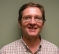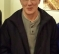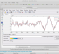## extract analysis and synthesis weighting filtersStarted by 3 months ago5 replieslatest reply 3 months ago107 views

I already posted my question on stackoverflow but hopefully I can get some comments/answers from DSP experts contributing to this forum too:

For regular FIR filters we can feed an impulse signal to get the coefficients, but how about filterbanks?

Let's say I have a uniformly modulated filterbank with hop size H and FFT size M, implemented as weighted overlap-add method . Is there a way to construct an input signal to extract the analysis and synthesis weights?

1. R. Crochiere and L. R. Rabiner, Multirate Digital Signal Processing, Englewood Cliffs, NJ: Prentice-Hall, 1983.
[ - ]
Reply by June 29, 2022Hello Drunken Monk!

The filter bank design methods I'm familiar with involve designing a prototype filter to meet the channel specifications.  The length and form of the filter are determined by the filter bank design.

I have that book; could you point me to the section you are talking about?  What type of filter bank?

Also, a little more information about what you are trying to do would be helpful.

Cheers,

Mark Napier

[ - ]
Reply by June 29, 2022Hello Mark,

Section 7.2.5, Fig. 19 and Fig. 20.

Sorry if my question was not clear; for the analysis operation the input signal is weighted by a window (h in Fig. 19) and then there is some time aliasing followed by DFT. I would like to know if it's possible to extract the coefficients of h.

Thanks

[ - ]
Reply by June 29, 20222nd time posting this.  Web page crapped out on me the first time.

OK, I think I understand.  You have a working black box.

You can do it with an input impulse if you can control the input time sequence perfectly say WRT a reset.

If M = length of the DFT and H is the number of sections the window is broken up into then the length of the filter h = H * M.

So a single input impulse will give you at least one non-zero output data set from the DFT.  Since each data set has M elements that means at least one element (probably most) will be non-zero.  The number of non-zero data sets from that single input impulse will tell you something about how much the input buffer is shifted for each DFT.

Another trick is recognizing that power in the time domain will equal power in the frequency domain. (Neglecting the scaling factor of the DFT).  So the sum of the power in all of the output bins in a single data set will equal the power of a single tap in h.  You could also just sum the squares of all the bins and set that equal to the square of the tap in h.

Shift the impulse over by one for each test run and that should give you all the taps in h.

Hope this makes some sense.

Mark Napier

[ - ]
Reply by June 29, 2022Monk, what's the link for your Stackoverflow post ?

[ - ]
Reply by June 29, 2022I guess it is this;